## Example Questions

### Example Question #1 : How To Find The Endpoints Of A Line Segment

There is a line defined by two end-points,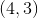and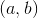.  The midpoint between these two points is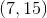.  What is the value of the point?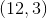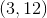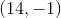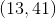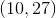Explanation:

Recall that to find the midpoint of two pointsand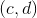, you use the equation: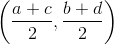.

(It is just like finding the average of the two points, really.)

So, for our equation, we know the following: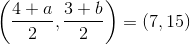You merely need to solve each coordinate for its respective value.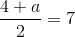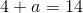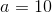Then, for the y-coordinate: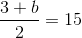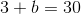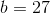Therefore, our other point is:### Example Question #1 : Midpoint Formula

There is a line defined by two end-points,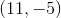and.  The midpoint between these two points is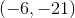.  What is the value of the point?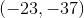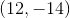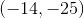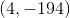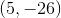Explanation:

Recall that to find the midpoint of two pointsand, you use the equation:.

(It is just like finding the average of the two points, really.)

So, for our equation, we know the following: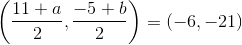You merely need to solve each coordinate for its respective value.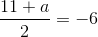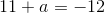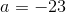Then, for the y-coordinate: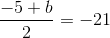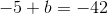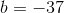Therefore, our other point is:### Example Question #3 : How To Find The Endpoints Of A Line Segment

What is the other endpoint of a line segment with one point that is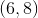and a midpoint of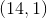?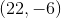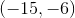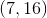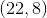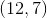Explanation:

Recall that the midpoint formula is like finding the average of theandvalues for two points.  For two pointsand, it is:For our points, we are looking for.  We know: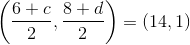We can solve for each of these coordinates separately:

X-Coordinate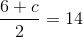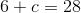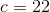Y-Coordinate: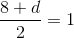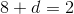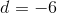Therefore, our point is### Example Question #4 : How To Find The Endpoints Of A Line Segment

What is the other endpoint of a line segment with one point that is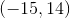and a midpoint of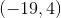?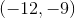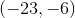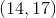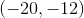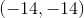Explanation:

What is the other endpoint of a line segment with one point that isand a midpoint of?

Recall that the midpoint formula is like finding the average of theandvalues for two points.  For two pointsand, it is:For our points, we are looking for.  We know: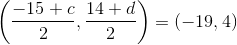We can solve for each of these coordinates separately:

X-Coordinate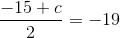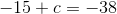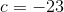Y-Coordinate: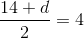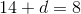Therefore, our point isTired of practice problems?

Try live online GRE prep today.